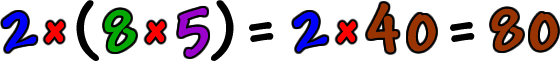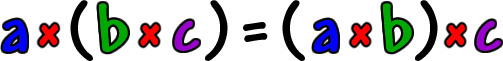How about trying the same thing with another set of numbers:Do we get the same thing when we multiply them...  even if we group the numbers differently?Yep.  Sure looks like it!

It's your turn to try it now...  Go ahead...  Pick any three numbers you want...  Group them one way and multiply them together...  Then, change the parenthesis and multiply them again!  I'll wait...

Did you get the same thing?  You always will.  When something happens like this in math, we make it a special rule called a "property."

Here's the property in all letters to get you ready for Algebra!

 The Associative Property of Multiplication:Where a, b and c are any real numbers.

Remember that you always do what's in the parenthesis first!  This rule just says that, when you are doing multiplication,  it doesn't matter which numbers you multiply first.  You can multiply the a and b first OR you can multiply the b and c first and you'll  get the same answer.

NOTE:  This does not work with division!